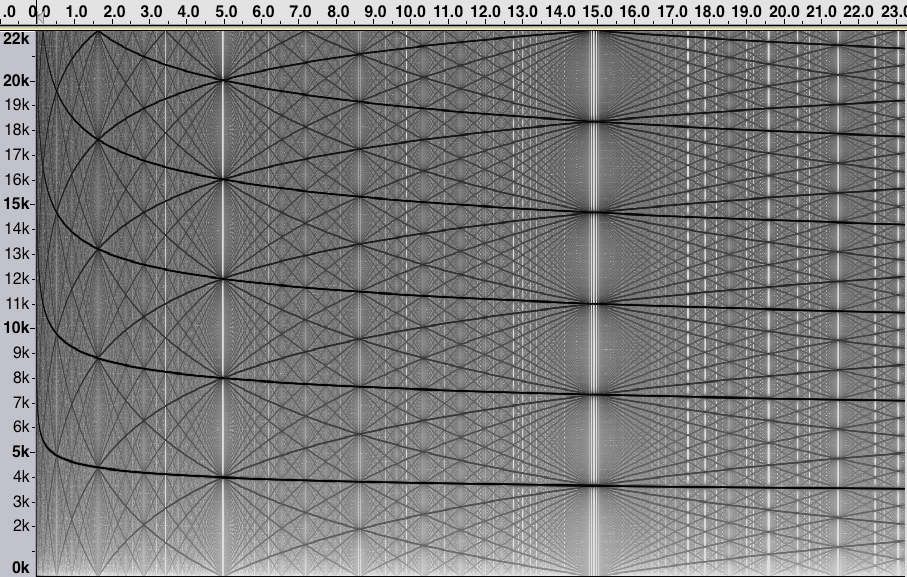Considering the fractional part of n log n is fruitful.

If, for some x=A, (x+1)log(x+1)-x log x is close to an integer k, then floor((x+1)log(x+1))-floor(x log x) = k for x near A. As a result, the waveform is periodic near this point, and the spectrogram shows a "convergence" to the equally-spaced frequencies that are multiples of 44100/k.

For example, (x+1)log(x+1)-x log x = 12 for x = 59873.64... Evaluating the sequence near here means the terms are about x log x = 658615, so at time about 658615/44100 = 14.93... seconds, and around this point, the terms of the sequence are equally spaced with constant difference 12. Hence, the spectrogram shows only multiples of 44100/12 = 3675 hz.

Likewise, for the same reason, there are similar phenomena at 4.99 seconds (multiples of 4009 hz), 1.65 seconds (multiples of 4410 hz), 0.54 seconds (multiples of 4900 hz), etc.

The spectrogram of A050504:OIES id description sound player creator A050504 floor of n log n download Hannah Claire Van Wyk

A similar, but less dramatic, phenomenon can be seen near places where (x+1)log(x+1)-xlog x is an integer plus 1/2.

For example, solving the equation (x+1)log(x+1)-x log x = 11.5 yields x=36315.00267539... . As a result, for n near 36315, we find that floor((n+1) log(n+1))-flor(n log n) alternates: 11, 12, 11, 12, 11, 12, ... . This means that, locally, the waveform has a period of 23, and so we see in the spectrograph multiples of 44100/23 = 1917.39.. hz at time approximately (36315 log 36315)/44100 = 8.65 seconds.

Similarly, around n=26902 (near 6.22 seconds), we see the differences between consecutive terms

....,12,11,11,11,11,12,11,11,11,11,12,11,11,11,11,12,11,11,11,11,12,11,11,11,11,12,...

So the sequences is behaving like a "periodic" sequence with period 12+11(4)=56, and the spectrogram shows a simple spectrum of multiples of 44100/56 = 787.5 hz. This is all a result of the fact that (x+1)log(x+1)-x log x = 12.2 has solution x = 26902.6860... .

Here is a proof that, for this sequence, the difference a_{n+1}-a_n = floor(log(n+1))+x, where x=1 or x=2.